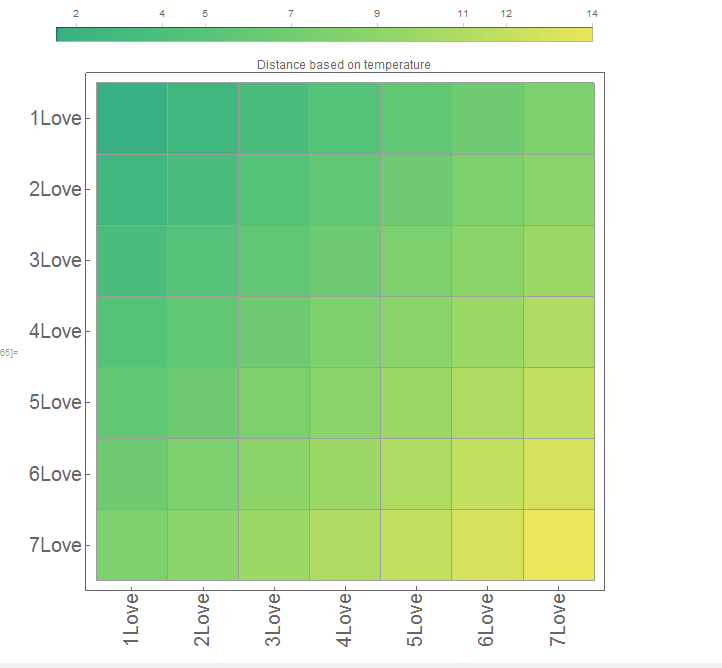# 距离矩阵可视化|Mathematica绘制可视化矩阵

• A+

## 代码介绍

``````
matPlot[dm_, capitals_] :=

Module[{},

MatrixPlot[dm,

FrameTicks -> {{Transpose[{Range[Length@capitals], capitals}],

None}, {Transpose[{Range[Length@capitals],

Rotate[#, \[Pi]/2] & /@ capitals}], None}}, Mesh -> True,

ColorFunction -> "BlueGreenYellow",

PlotLegends -> Placed[Automatic, Above],

PlotLabel -> "Distance based on temperature", ImageSize -> Large]

]
``````

### 使用介绍

``````
(*dm是矩阵,capitals是坐标对应的标签*)

dm = Table[i + j, {i, 1, 7}, {j, 1, 7}];

cap = Table[Style[ToString[i] <> "Love", 20], {i, 1, 7}];
``````

``````
matPlot[dm, cap]
``````• 微信公众号
• 关注微信公众号
•• QQ群
• 我们的QQ群号
•